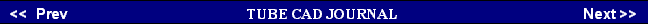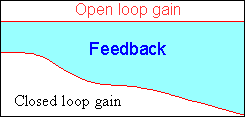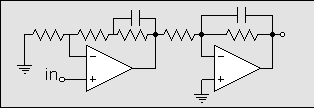Now +60 dB equals a gain of 1000. This amount of gain could be had from cascading two +30 dB (gain of 31.6) grounded-cathode stages, which is easily achieved with two 12AX7s or even two 12AT7 triodes, but not by two 12AU7s or two 6DJ8s, as their amplification factors are too low.      If we increase the desired gain to +80 dB (a gain of 10,000), then even two 12AX7s will not do, as we never realize the full potential gain implied by a triode's mu in a grounded-cathode amplifier, except when it is loaded by a constant current source and works into no other load impedance. One work-around is to use a cathode follower after each grounded-cathode amplifier, but this approach was seldom if ever taken.        So here is the dilemma: we do not want to exceed two gain stages and yet we want more gain. One solution has been to use at least one cascode stage in the mix. The cascode circuit has the very desirable attribute of realizing a gain in excess of the mu of the triode used. (This plan was beautifully implemented in Audio Research's SP-10 and MFA's MC Reference preamp.) Pentode-based circuits and hybrid circuits can also realize a much larger gain than can be developed by the triodes alone.         Still another solution can be gleaned from some solid-state phono preamps: forgo the single-all-encompassing-equalizing feedback loop around the entire preamp stage. In other words,  split the equalization curve into its sub-curves and use two gain stages, each with its own equalizing feedback loop. For example, the fist stage can produce the 50-Hz to 500-Hz part of the RIAA equalization curve, while the second stage can yield the 2122-Hz low-pass function of the curve. This arrangement greatly unburdens each amplifier stage and allows for the realization of a much greater total gain.
 Active Equalization       Active RIAA equalization means feedback equalization: the frequency response is tailored to fit that of the RIAA curve by varying the amounts of feedback returned to the input. The advantage of using feedback is consistency. Each channel will track the other to a very great degree in spite of aging parts or circuit wiring dissimilarities, as the feedback tends to iron everything out. The disadvantage is that, because it is active, the circuit can more readily suffer from input voltage overloads and the preamp must have voltage gain far in excess of nominal +40 dB usually specified, as the feedback uses the excess gain to force the output to conform to the desired curve. Another problem is potential instability, as each coupling capacitor and gain stage add some phase shift.      Since the bass frequencies must be amplified +20 dB higher than the 1-kHz center frequency, the +20 dB must be added to the +40 dB of gain, yielding +60 dB of total gain. On top of this +60 dB an additional 20 to 30 dB of gain might be added to feed the feedback mechanism. Thus, we need more gain, but we cannot risk adding more gain stages, as each coupling capacitor and Miller effect capacitance adds some phase shift, which can reverse the phase of the fed back signal, creating an oscillator, not an amplifier.      In the absence of this extra gain, the varying amount of frequency dependent feedback can result in looser bass reproduction because of smaller amount of feedback at low frequencies and possibly a pinched, compressed high frequency playback due to excessive feedback ratios at high frequencies since a 20-kHz signal is attenuated by -40 dB relative to a 20-Hz signal. In other words,  a preamp with only +60 dB of open-loop gain will have zero feedback at 20-Hz and 40 dB of feedback at 20-kHz.60dB40dB20dB